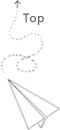# Tips of bit index and bitmask (By solution)

Some notes about the bit index used in the programming.

Using the bitset as the index can save the size of the key a lot, for example, one unsigned int (4byte) can represent 32bit, there are 2^32 key in totoal.

The bit set case is also conceneint to express the case associated with the binary tree. We can increase the length of the tree by adding one digit at the end of the key string, which can simplify the programming a lot.

In cpp, there is bitset in the std library, which represents fixed length of n bit, we can set specifc bit as 1 or 0.

### bit index to int (tree based index to flattern index)

When we go through a binary tree (assuming there are 8 depth, which is 256 keys), if the value assocaited with key sataisfy requirements, we process it.

For this example, the output is 1,3,7,15,31,63,127,255, we actually doing a selection here and finding a path by go through each level of the binary tree. (assuming the leaf node store the actual value from 0 to 255), we do the selection by gradually checking the associated value for each bit is 0 or 1.

### int to bit index (flattern index to the tree based index)

in this case, we use the integer as a flatten index, then we checking for each bit, if it is zero or one, if it is zero, it goes to specific branch, if it is 1, then it goes to another branch. Using 0 and 1 to decide the positive case or the negative case.

For this case, we use the flatten index 7 as the input, the output is

The first three digits are positive for 7 and other digits are negative.

### using bitmask to replace the visit array

Typical operation includes set a specific position to 1 or 0. The initilization is just to init as a number, such as int or long int, which depends on number of bit. For the int, there is 4 byte, so it can represents 32 bit, the flag index for 32 bit, long int number can represent 64 bit.

This can replace the visited[index]=1.

This can replace the visited[index]=0.

This can check the existance of specific bit to check if it is 0 or 1.

By doing this, we can improve the algorithm’s time and even use this bitmask as a index for dp based memorization.

## 推荐文章